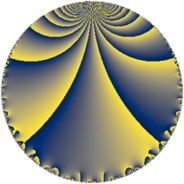# Properties

 Label 32.4.bLevel $32$ Weight $4$ Character orbit 32.b Rep. character $\chi_{32}(17,\cdot)$ Character field $\Q$ Dimension $2$ Newform subspaces $1$ Sturm bound $16$ Trace bound $0$

# Related objects

## Defining parameters

 Level: $$N$$ $$=$$ $$32 = 2^{5}$$ Weight: $$k$$ $$=$$ $$4$$ Character orbit: $$[\chi]$$ $$=$$ 32.b (of order $$2$$ and degree $$1$$) Character conductor: $$\operatorname{cond}(\chi)$$ $$=$$ $$8$$ Character field: $$\Q$$ Newform subspaces: $$1$$ Sturm bound: $$16$$ Trace bound: $$0$$

## Dimensions

The following table gives the dimensions of various subspaces of $$M_{4}(32, [\chi])$$.

Total New Old
Modular forms 16 4 12
Cusp forms 8 2 6
Eisenstein series 8 2 6

## Trace form

 $$2 q + 16 q^{7} - 2 q^{9} + O(q^{10})$$ $$2 q + 16 q^{7} - 2 q^{9} - 112 q^{15} - 28 q^{17} + 304 q^{23} + 26 q^{25} - 448 q^{31} + 168 q^{33} + 560 q^{39} - 140 q^{41} - 672 q^{47} - 558 q^{49} + 336 q^{55} + 392 q^{57} - 16 q^{63} + 1120 q^{65} + 144 q^{71} - 588 q^{73} + 928 q^{79} - 1510 q^{81} - 1680 q^{87} + 532 q^{89} + 784 q^{95} + 1988 q^{97} + O(q^{100})$$

## Decomposition of $$S_{4}^{\mathrm{new}}(32, [\chi])$$ into newform subspaces

Label Dim $A$ Field CM Traces $q$-expansion
$a_{2}$ $a_{3}$ $a_{5}$ $a_{7}$
32.4.b.a $2$ $1.888$ $$\Q(\sqrt{-7})$$ None $$0$$ $$0$$ $$0$$ $$16$$ $$q-\beta q^{3}-2\beta q^{5}+8q^{7}-q^{9}+3\beta q^{11}+\cdots$$

## Decomposition of $$S_{4}^{\mathrm{old}}(32, [\chi])$$ into lower level spaces

$$S_{4}^{\mathrm{old}}(32, [\chi]) \cong$$ $$S_{4}^{\mathrm{new}}(8, [\chi])$$$$^{\oplus 3}$$